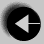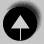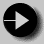## The for Loop

The for loop works well where the number of iterations of the loop is known before the loop is entered. The head of the loop consists of three parts separated by semicolons.

• The first is run before the loop is entered. This is usually the initialisation of the loop variable.
• The second is a test, the loop is exited when this returns false.
• The third is a statement to be run every time the loop body is completed. This is usually an increment of the loop counter.

The example is a function which calculates the average of the numbers stored in an array. The function takes the array and the number of elements as arguments.

```
float average(float array[], int count)
{       float total = 0.0;
int i;

for(i = 0; i < count; i++)
total += array[i];

return(total / count);
}```

The for loop ensures that the correct number of array elements are added up before calculating the average.

The three statements at the head of a for loop usually do just one thing each, however any of them can be left blank. A blank first or last statement will mean no initialisation or running increment. A blank comparison statement will always be treated as true. This will cause the loop to run indefinitely unless interrupted by some other means. This might be a return or a break statement.

It is also possible to squeeze several statements into the first or third position, separating them with commas. This allows a loop with more than one controlling variable. The example below illustrates the definition of such a loop, with variables hi and lo starting at 100 and 0 respectively and converging.

```
for (hi = 100, lo = 0; hi >= lo; hi--, lo++)```

The for loop is extremely flexible and allows many types of program behaviour to be specified simply and quickly.Go to The do while LoopGo to IndexGo to the break Statement